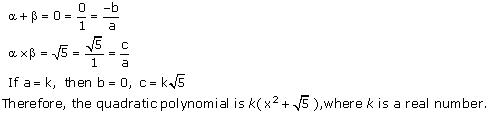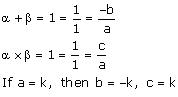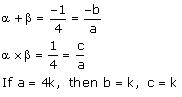# NCERT Solutions for Class 10 Maths Chapter 2 Polynomials Ex 2.2

NCERT Solutions for Class 10 Maths Chapter 2 Polynomials Ex 2.2 are part of . Here are we have given Chapter 2 Polynomials Class 10 NCERT Solutions Ex 2.2.

 Board CBSE Textbook NCERT Class Class 10 Subject Maths Chapter Chapter 2 Chapter Name Polynomials Exercise Ex 2.2 Number of Questions Solved 2 Category NCERT Solutions

## NCERT Solutions for Class 10 Maths Chapter 2 Polynomials Ex 2.2

Page No: 33

Question 1. Find the zeroes of the following quadratic polynomials and verify the relationship between the zeroes and the coefficients.
(i) x2 – 2x – 8
(ii) 4s2 – 4s + 1
(iii) 6x2 – 3 – 7x
(iv) 4u2 + 8u
(v) t2 – 15
(vi) 3x2 x – 4

Solution:So, the zeroes of x² – 2x – 8 are 4 and -2.Concept Insight: The zero of a polynomial is that value of the variable which when substituted in the polynomial makes its value 0. When a quadratic polynomial is equated to 0, then the values of the variable obtained are the zeroes of that polynomial. The relationship between the zeroes of a quadratic polynomial with its coefficients is very important. Also, while verifying the above relationships, be careful about the signs of the coefficients.

Question 2. Find a quadratic polynomial each with the given numbers as the sum and product of its zeroes respectively.
(i) 1/4, -1
(ii) √2, 1/3
(iii) 0, √5
(iv) 1,1
(v) -1/4,1/4
(vi) 4,1

Solution:
(i)    Let the required polynomial be  ax² + bx + c, and let its zeroes α and βIf a = 4k, then b = -k, c = -4k  Therefore, the quadratic polynomial is k(4 x 2 – x – 4), where k is a real number .
(ii)     Let the polynomial be  ax² + bx + c, and let its zeroes be α and β(iii)    Let the polynomial be  ax² + bx + c, and let its zeroes be α and β(iv)    Let the polynomial be  ax² + bx + c, and let its zeroes be α and β
Therefore, the quadratic polynomial is k(x² – x + 1), where k is a real number.(v)    Let the polynomial be ax² + bx + c, and its zeroes be α and β
Therefore, the quadratic polynomial is k(4x² + x + 1),where k is a real number .
(vi)    Let the polynomial be ax² + bx + c.Therefore, the quadratic polynomial is k(x² – 4x + 1), where k is a real number.

Concept Insight:  Since the sum and product of zeroes give 2 relations between three unknowns so we assign a value to the variable a and obtain other values.
Alternatively, If the sum and the product of the zeroes of a quadratic polynomial is given then polynomial is given by, where k is a constant. And the simplest polynomial will be the one in which k = 1.

We hope the NCERT Solutions for Class 10 Maths Chapter 2 Polynomials Ex 2.2 help you. If you have any query regarding NCERT Solutions for Class 10 Maths Chapter 2 Polynomials Ex 2.2, drop a comment below and we will get back to you at the earliest.